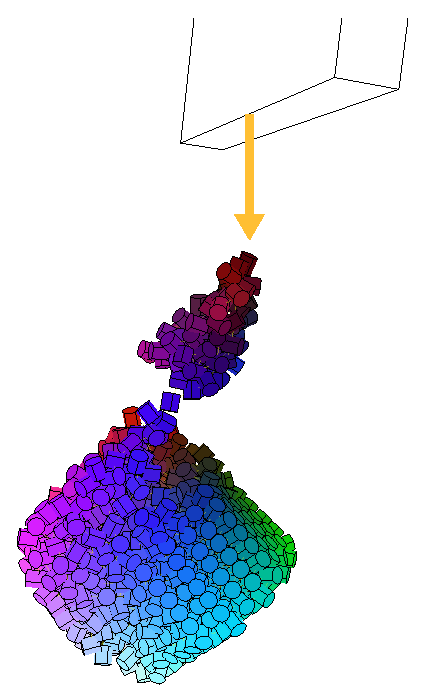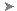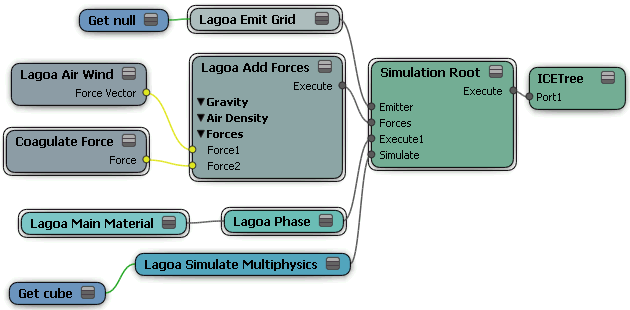Forces make particles and simulated deformed objects move according to different types of natural (or unnatural) forces. Each simulated object can have multiple forces applied to it.The blob tries to stick to the collision object, but gravity overcomes it, pulling it down.

As with other ICE simulators, the Lagoa simulator does its force calculations as if a point's mass has a value of 1 (see ICE Particle Mass [ICE Particle Simulations] for more information).

The Lagoa Air Density node is the main forces node that you need to have in a Lagoa ICE tree. It is the hub into which you can plug other force nodes, similar to the Add Forces node.

Once you have the Lagoa Add Forces node plugged in to the Lagoa ICE tree, you can plug the Lagoa Air Wind Force into it, as well as any of the other ICE force nodes (see ICE Forces [ICE Fundamentals]). The order in which the forces are plugged into the Lagoa Add Forces node is not important because the forces are simply added together.

The Lagoa Add Forces node has gravity and air density forces built into it, and are active by default. When working in a simulation environment, it's recommended that you set the gravity to the value you want, and then leave it like that so that your environment has a base line for calculations.

To apply forces to a Lagoa simulation

1. Create a Lagoa ICE tree with the required nodes as described in Creating a Lagoa Effect from Scratch.

2. Plug the Lagoa Add Forces node into the Forces port of the Simulation Root node, or into a port on the ICETree node.

3. In the Lagoa Air Density property editor, set the parameters.

TipTo open the Lagoa Add Forces property editor without having the ICE tree open, select the Lagoa point cloud or polygon mesh and choose ParticlesForceLagoa ParticlesInspect Gravity or Inspect Air Density from the ICE toolbar.
4. Plug other forces into the Lagoa Add Forces ports as you like. You can do any of the following:

• Plug the Material Type into a Force port.

• Plug any of the ICE forces in the Particles > Forces group into a Force port.

• Select the Lagoa-simulated object and choose any of the ParticlesForces commands from the ICE toolbar to apply ICE forces. These commands work only if the Simulation Root node is plugged into the ICE tree.